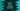# Python program to print a triangle using star## Python 3 program to print a Triangle using star :

In this tutorial, we will learn how to print a triangle using star (* _). You can change it to any other characters if you want. Printing a symbol works the same for any programming language. You can use the same logic on any other programming language like Java, R, C, C++ _etc. to get the same output.

I will also teach you later how to solve any problems like this one.

The program will take the height of the triangle as an input from the user. It will print out the triangle using star(_* _). You can customize this program to print the triangle using any other character.

### Printing triangle using star :

``````#program 1
height = int(input("Enter the height of the triangle : "))

for i in range(height,0,-1):
print(i* ' ' + (height+1-i) * '*')

#program 2
height = int(input("Enter the height of the triangle : "))

for i in range(height,0,-1):
print(i* 'k' + (height+1-i) * '*')``````

#### The program to print triangle using star is as below :

Let’s check one example of how it will print :

We are using only one single print statement to write down the whole logic of the program. But how is it working? To understand the concept, let me do some modification to the program :

You can see the only thing we have changed is that we are printing out ‘k’ instead of space in the above program. It will result like below :

#### In the above pattern :

1. k _’ is printed instead of a space ‘ ’. Think ‘k’ as _space.
2. For the first line, ‘k _’ is printed for ‘height ’ times and ‘* ’ for _1 time. The height of the triangle is 5, so k is printed five times. In our original program, space was printed instead of ‘_k _‘.
3. For the second line, ‘k _’ is printed for ‘height – 1’ times and ‘* ’ for _2 times. ’k _’ is printed _four times and star is printed two times.
4. For the third line, ‘k _’ is printed for ‘height – 2 ’ times and ‘* ’ for _3 times. So, the number of times ’k _’ is printed is decreased by one on each step. For the first line, _k is printed for height times or 5 times, for the second line, k is printed for 4 times, for the third time, it is printed for 3 times etc.

Similarly, the character or ’* _’ is printed for _one time in the first line, it is printed two times in the second line, three times in the third line etc.

We need only the height of the triangle. Based on its value, we can print it out using the above steps.

Now, let’s try to understand the program :

1. We have a ‘for _’ loop that runs (height) times. ‘i ’ is the value in this loop. ‘i ’ will run from the value (height + 1_) to 1. Each time it will be decremented by 1.
2. Inside the loop, first space ‘ ’ is printed for ‘i _’ times and then ‘* ’ is printed for (height + 1 -i _) times. Each line is printed on a new line. That’s it. You can write down each step of the loop to understand the program better.

### Conclusion :

In this tutorial, we have learned how to print a triangle using star in python. You can modify the program to print the triangle with any other character you want or you can also print the triangle using numbers or characters. Try to run the above example and drop one comment below if you have any queries.